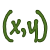Games
Problems
Go Pro!

# Non-Linear

Pro Problems > Math > Algebra > Equations > Systems of Equations > Non-Linear

# Featured Pro ProblemsFind the sum of x and y, if the following quadratic equations in x and y are true.Solve the system of equations which involves a product of variablesSolve the system of equations in x and y - one of the equations is cubic!Solve the quadratic system of equations.Two quadratic equations in two variables - solve for m and nA system of equations involving a product of the variablesA system of three equations in three unknowns, involving a bunch of sumsA second degree system problem that is much simpler than it looks, if you find the right approach

# Full Directory Listing

Cubic and Linear, Linear and Quadratic, Mary and Laura's Cookies, One Equation, Two Variables, Product of X and Y, Quadratic System, Quadratic System, Sum and Product System, Sum of X and Y, System with a Product, System with Radical, To Sum It Up, X and Y Quadratics, X and Y SystemLike us on Facebook to get updates about new resources